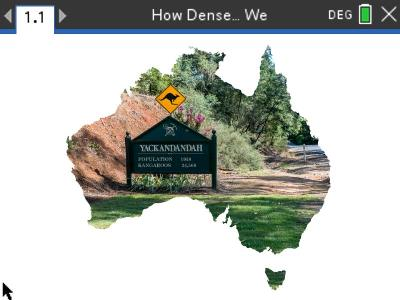Activities

•30 Minutes

4.5

How dense are weActivity Overview

In this activity students use scientific notation to compare populations, state land areas and the corresponding population density.

Objectives

• Review of computation: order of operations, directed numbers, scientific notation, estimation, exact and approximate answers.

Vocabulary

• Scientific notation
• Population
• Density
• Significant figures